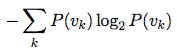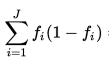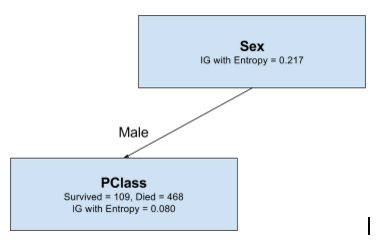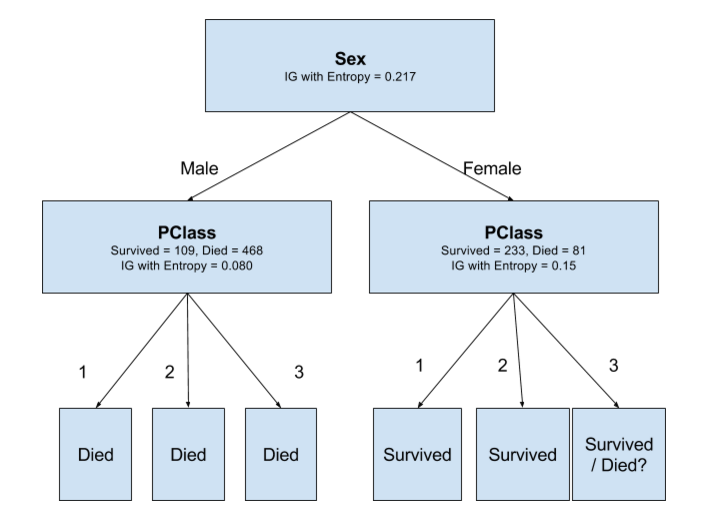# DECISION TREES ASSIGNMENT

Akhil Stanislavose

September 20, 2016

# CODE

There is a lot of code, mostly repetitive, which I could not put on the slides as the lines are long and it will be difficult to read off the slides. So I have put the entire code on an IPython Notebook which can be accessed at

## Exploring the Dataset

• How many examples?
• How many positives (survived)?
• How many negatives (did not survive)?

# RUBY

## OPEN CSV IN RUBY

`````` require 'csv'

```` dataset = CSV.parse(csv, :headers => true)`` puts dataset.count`` # => 891````

## FILTERING RECORDS

How may died and survived?

``````died = dataset.select { |e| e['survived'] == '0' }.count
# => 549

survived = dataset.select { |e| e['survived'] == '1' }.count
# => 342``````

# GINI

## ENTROPY

``````  def entropy(probablities)
probablities.reduce(0.0) do |sum,p| ``````      if p > 0
sum += p * Math.log2(p)````      else``        sum += 0````      end
end * -1
end
``````## GINI

``````  def gini(probablities)
probablities.reduce(0.0) do |sum,p|
sum += p * (1 - p)
end
end``````## PURITY

``````
def purity(mixtures, &block)
purity = mixtures.reduce(0.0) do |sum,m|
size = m.reduce(:+).to_f
measure = yield(m.collect { |n| n/size })
sum += size > 0 ? size * measure : 0
end
purity / mixtures.flatten.reduce(:+)
end

``````

## ENTROPY & GINI OF DATASET

``````
died     = dataset.select { |e| e['survived'] == '0' }.count
survived = dataset.select { |e| e['survived'] == '1' }.count

dataset_entropy = purity([[died,survived]]) { |ps| entropy(ps) }
dataset_gini    = purity([[died,survived]]) { |ps| gini(ps) }

puts "Entropy of dataset = #{dataset_entropy}"
puts "Gini of dataset    = #{dataset_gini}"

# Entropy of dataset = 0.9607079018756469
# Gini of dataset    = 0.4730129578614427

``````

### IG after gender split using Entropy

0.2176601066606143

### IG after gender split using Gini

0.13964795747285225

### IG after pclass split using Entropy

0.08383104529601149

### IG after pclass split using Gini

0.05462157677138346

### IG after embarked split using Entropy

0.024047090707960517

### IG after embarked split using Gini

0.015751498294317823

# DECISION TREE

## ROOT NODE# SPLIT MALES BY

## PCLASS

FIND IG IN EACH CASE

## TREESO WE USE

# PLURALITY-VALUE

The function PLURALITY-VALUE selects the most common output value among a set of examples, breaking ties randomly.

## TREEDIED(P1) > SURVIVED(P1)
DIED(P2) > SURVIVED(P2)
DIED(P3) > SURVIVED(P3)

# SPLIT FEMALES BY

## PCLASS

FIND IG IN EACH CASE

## TREE## USE PLURALITY-VALUE## PERFORMANCE

If we look at the tree I have made it is very evident that

## else if male he dies.

For simplicity if we use our entire training set as the test set,

and

## 314 will live

But from the data we know that actually only

and

# WEKA

## FIN.

Questions?

#### TITANIC DATASET

By akhil stanislavose

• 803